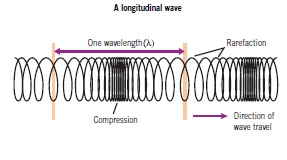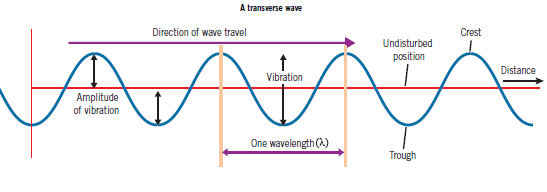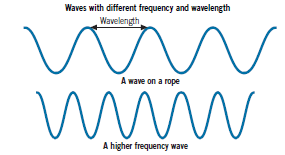## Title

Describing Waves
Quick revise

Transverse and Longitudinal Waves

A wave is a vibration or disturbance transmitted through a material (a medium) or through space. Waves transfer energy and information from one place to another, but they do not transfer material.

A transverse wave has vibrations at right angles (perpendicular) to the direction of travel. The wave has crests and troughs.

Examples include water waves, waves on strings or rope, light and other electromagnetic waves.

A longitudinal wave has vibrations parallel to the direction of wave travel. It has compressions and between these are stretched parts called rarefactions.

Examples include sound waves, ultrasound waves, which are sound waves with frequency greater than 20 kHz, and waves along a springThere are two types of seismic waves called P-waves and S-waves:

• P-waves are longitudinal waves that travel through solid or liquid rock. They travel faster than S-Waves.
• S-waves are transverse waves that can only travel through solid materials in the Earth.

Wave Properties

Amplitude is the maximum displacement (change in position) from the undisturbed position.

Wavelength (λ) is the distance in metres from any point on the wave to an exactly similar point.

Frequency is the number of waves that pass a point in one second. This depends on how fast the source of the waves is vibrating. The
frequency is usually expressed in hertz (Hz) where one Hz is one cycle (wave) per second.

Example: Four waves pass a point in one second. The frequency = 4 Hz.

Example: A wave takes two seconds to pass a point. The frequency = 0.5 Hz

Wave speed depends on the medium that the wave is travelling through:

distance wave travels = wave speed × time (d = v × t )

Example: A water wave travels at 5 cm/s. In 4 s it travels d = 5 cm/s × 4 s = 20 cm.

The wave equation relates the wavelength and frequency to the wave speed. For all waves: wave speed = frequency × wavelength (v = f λ where f is in Hz, λ is in m, v is in m/s).

Example: Water waves with wavelength λ = 10 cm and frequency f = 4 Hz have a speed v = 10 cm × 4 Hz= 40 cm/s.Sound Waves

For sound and ultrasound waves:

• The pitch of the sound is the frequency of the vibrations.
• The loudness of the sound depends on the amplitude of the vibrations

Sound travels much faster in solids than in liquids and faster in liquids than in gases.

This video explains the pitch and frequency of sound

Rate: